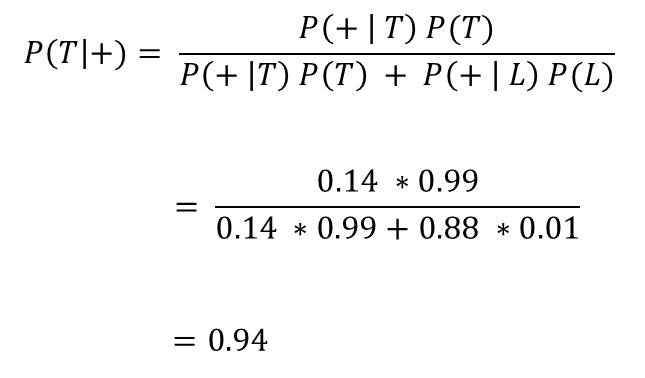# Conditional Probability – Application of Bayes’s Rule

In the previous lesson(Introduction to Bayes’ Rule), we saw how Bayes’ Rule can be applied to medical diagnosis.

So in this chapter, we would see how Bayes’ Rule applies to interrogation.  Let’s take an example.

The Polygraph Example

A polygraph test ( also called a lie-detector test) is normally administered to crime suspects during investigation.

Let + denote the event that the polygraph give a positive result (indicating that the suspect is lying)

Let denote the event that the polygraph gives a negative result(indicating that that suspect is telling the truth)

Let T denote the event that the suspect is telling the truth

Let L denote the event that the suspect is lying

From studied carried out, it is found out that:

P(+ | L) = 0.88 (meaning that the polygraph detects liars with an accuracy of 88%

It follows that, P(- | L) = 0.12 (this is false negative and follows from P(- | L) = 1 – P(+ | L)

Also P(- | T) = 0.86 (meaning that the polygraph detects when truth is told with accuracy of 86%

It also follow that P(+ | T) = 0.14 (this is false positive)

Assuming that on a particular question, almost all suspects have no reason to lie and so P(T) = 0.99. Meaning that P(L) = 0.1.

If a suspect produces a positive test result on the polygraph. What is the probability that the the this is a false positive (the polygraph is incorrect) and the suspect is telling the truth?

This can be evaluated using Bayes’ RuleInterpretation

This means that there is 94% chance that the polygraph is incorrect. Or 94% of the persons who are detected as lying were actually telling the truth.

This is why the results of Polygraph tests are not admissible in court. They are only used to assist the investigation process.#### kindsonthegenius

Kindson Munonye is currently completing his doctoral program in Software Engineering in Budapest University of Technology and Economics

View all posts by kindsonthegenius →

## One thought on “Conditional Probability – Application of Bayes’s Rule”

1.RonnyBig says:

Hi. I have checked your kindsonthegenius.com and i see you’ve got some duplicate content so
probably it is the reason that you don’t rank high in google.
But you can fix this issue fast. There is a tool that rewrites content like human, just search in google: miftolo’s tools